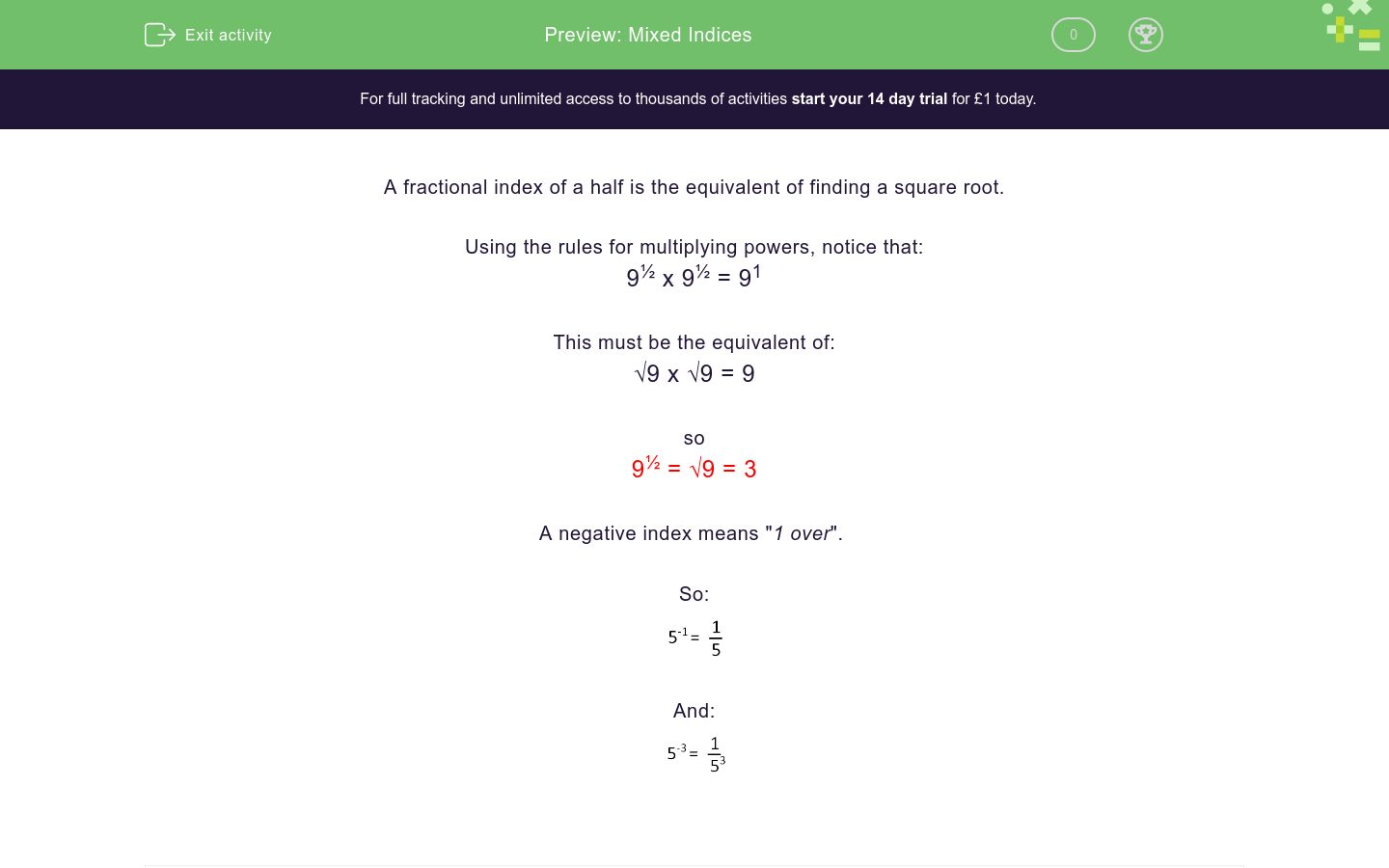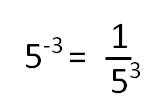# Mixed Indices

In this worksheet, students understand and use fractional and negative indices.Key stage:  KS 4

Curriculum topic:  Number

Curriculum subtopic:  Calculate with Roots and Integer (Fractional) Indice

Difficulty level:\$appendString = '&new=1'; \$_SESSION['guest_aId'] = rand(); \$urlToRedirect = FRAMEWORK_PATH . 'preview/worksheet/introduction?aId=' . \$_SESSION['guest_aId'] . '&wId=' . \$wsData['id'] . '&usertype=guest' . \$appendString; ?>

### QUESTION 1 of 10

A fractional index of a half is the equivalent of finding a square root.

Using the rules for multiplying powers, notice that:

9½ x 9½ = 91

This must be the equivalent of:

√9 x √9 = 9

so

9½ = √9 = 3

A negative index means "1 over".

So:And:Select the answer which is equal to:

5-1

-5

0.2

Select the answer which is equal to:

64½

8

64½

16

Select the answer which is equal to:

2-1

1

½

-21

Select the answer which is equal to:

4

-2

½

-4.5

Select the answer which is equal to:

25

0.2

-√12.5

√25

Select the answer which is equal to:

81½

40.5

9

81½

Select the answer which is equal to:

4-2

1

-8

1/16

Select the answer which is equal to:

10000

1000

0.01

10½

Select the answer which is equal to:

1000

33.3

100

1/√1000

Select the answer which is equal to:

12100½

1100

√121

110

• Question 1

Select the answer which is equal to:

5-1

CORRECT ANSWER
0.2
• Question 2

Select the answer which is equal to:

64½

CORRECT ANSWER
8
• Question 3

Select the answer which is equal to:

2-1

CORRECT ANSWER
½
• Question 4

Select the answer which is equal to:

4

CORRECT ANSWER
½
• Question 5

Select the answer which is equal to:

25

CORRECT ANSWER
0.2
• Question 6

Select the answer which is equal to:

81½

CORRECT ANSWER
9
• Question 7

Select the answer which is equal to:

4-2

CORRECT ANSWER
1/16
• Question 8

Select the answer which is equal to:

10000

CORRECT ANSWER
0.01
• Question 9

Select the answer which is equal to:

1000

CORRECT ANSWER
1/√1000
• Question 10

Select the answer which is equal to:

12100½

CORRECT ANSWER
110
---- OR ----

Sign up for a £1 trial so you can track and measure your child's progress on this activity.

### What is EdPlace?

We're your National Curriculum aligned online education content provider helping each child succeed in English, maths and science from year 1 to GCSE. With an EdPlace account you’ll be able to track and measure progress, helping each child achieve their best. We build confidence and attainment by personalising each child’s learning at a level that suits them.

Get started#### Similar activities you may like

Start your £1 trial today.
Subscribe from £10/month.